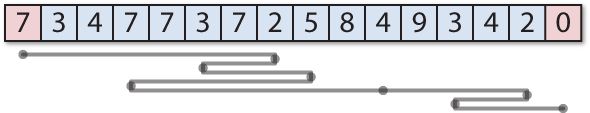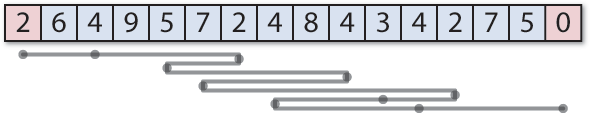## Tweetmaze solution

1st January 2016

Here's my solution to the Tweetmaze challenge, to produce the shortest possible Lisp program that solves a Tweetmaze. It implements a breadth-first-search using a queue of the previous routes taken:

```(defun solve-tweetmaze (maze &optional (queue '((0))))
(let ((goal (1- (length maze))))
(if (= (caar queue) goal)
(reverse (car queue))
(let* ((top (pop queue))
(position (first top))
(right (+ position (nth position maze)))
(left (- position (nth position maze))))
(when (>= left 0) (setq queue (push (cons left top) queue)))
(when (<= right goal) (setq queue (push (cons right top) queue)))
(solve-tweetmaze maze (sort queue #'< :key #'length))))))```

The routine solve-tweetmaze takes two parameters: the maze to be solved, as a list of numbers, and the queue of paths taken so far, sorted with the shortest path at the front. It returns the solution, as a list of positions. Initially the queue contains just the starting position, (0).

If the last cell visited by the shortest path is the goal, the routine returns the path. Otherwise it pops the shortest path from the front of the queue, and adds the two new paths to the queue corresponding to going left and right from the current position.

Finally it calls solve-tweetmaze again with the queue sorted to bring the shortest path to the front.

### First Tweetmaze

Here's an example of it solving the first Tweetmaze I gave last month:

```CL-USER > (solve-tweetmaze '(7 3 4 7 7 3 7 2 5 8 4 9 3 4 2 0))
(0 7 5 8 3 10 14 12 15)```

Here's a graphical representation of this solution:### Second Tweetmaze

Here's an example of it solving the second Tweetmaze I gave last month:

```CL-USER > (solve-tweetmaze '(2 6 4 9 5 7 2 4 8 4 3 4 2 7 5 0))
(0 2 6 4 9 5 12 10 7 11 15)```

Here's a graphical representation of this solution:### Limitations

My routine is very inefficient, and has the following limitations:
• It doesn't check for positions in the maze that have already been visited.
• If there are several solutions of the same length it only finds the first one.
• It doesn't detect if there's no solution.
• Instead of sorting the queue each time it would be more efficient to keep the queue sorted by pushing the two new paths to the correct position in the queue.

For a solution that fixes these limitations see Javier Olaechea's solution, tweet-maze-general.lisp.

On thinking about my earlier solution I realised that, because we're always extending the shortest path by one, at any time the queue only contains paths of length n and n+1. We can therefore simply append the new paths onto the end of the queue, avoiding the need for the call to sort:

```(defun solve-tweetmaze (maze &optional (queue '((0))))
(let ((goal (1- (length maze))))
(if (= (caar queue) goal)
(reverse (car queue))
(let* ((top (pop queue))
(position (first top))
(right (+ position (nth position maze)))
(left (- position (nth position maze))))
(when (>= left 0) (setq queue (nconc queue (list (cons left top)))))
(when (<= right goal) (setq queue (nconc queue (list (cons right top)))))
(solve-tweetmaze maze queue)))))```

For efficiency I'm using the destructive version of append, nconc, since we are replacing the original queue.